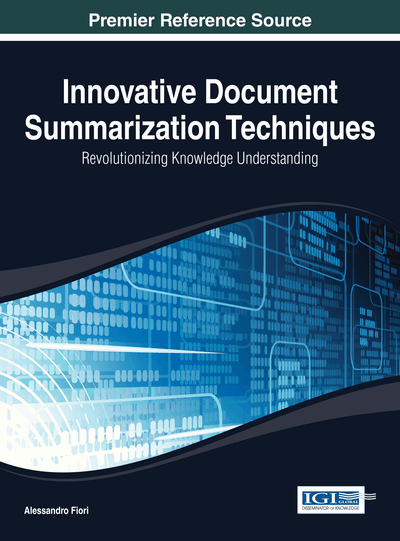# Efficient Summarization with Polytopes

Marina Litvak (Shamoon College of Engineering, Israel) and Natalia Vanetik (Shamoon College of Engineering, Israel)
DOI: 10.4018/978-1-4666-5019-0.ch003

## Abstract

The problem of extractive summarization for a collection of documents is defined as the problem of selecting a small subset of sentences so that the contents and meaning of the original document set are preserved in the extract in best possible way. In this chapter, the authors present a linear model for the problem of extractive text summarization, where they strive to obtain a summary that preserves the information coverage as much as possible in comparison to the original document set. The authors measure the information coverage in terms and reduce the summarization task to the maximum coverage problem. They construct a system of linear inequalities that describes the given document set and its possible summaries and translate the problem of finding the best summary to the problem of finding the point on a convex polytope closest to the given hyperplane. This re-formulated problem can be solved efficiently with the help of linear programming. The experimental results show the partial superiority of our introduced approach over other systems participated in the generic multi-document summarization tasks of the DUC 2002 and the MultiLing 2013 competitions.
Chapter Preview
Top

## Introduction

Automated text summarization is an active field of research attracting much attention from both academic and industrial communities. Summarization is important for IR systems since it helps to access large repositories of textual data efficiently by identifying the essence of a document and indexing a repository. Also, summarization provides end users shorter versions of the original documents that retain their most important points and, as result, saves time user needs for getting conclusions and decisions. Taxonomically, we distinguish between single-document, where a summary per single document is generated, and multi-document, where a summary per cluster of related documents is generated, summarization. Also, we distinguish between automatically generated extract— the most salient fragments of the input document/s (e.g., sentences, paragraphs, etc.) and abstract— re-formulated synopsis expressing the main idea of the input document/s. Since generating abstracts requires a deep linguistic analysis of the input documents, most existing summarizers work in extractive manner (Mani and Maybury, 1999). Moreover, extractive summarization can be applied to cross-lingual/multilingual domains (Litvak et al., 2010).

In this paper we deal with the problem of extractive summarization. Our method can be generalized for both single-document and multi-document summarization. Since the method includes only very basic linguistic analysis, it can be applied to multiple languages.

Formally speaking, in this paper we introduce:

• A novel text representation model expanding a classic Vector Space Model (Salton et al., 1975) to Hyperplane and Half-spaces;

• A distance measure between text and information coverage we wish to preserve;

• A re-formulated extractive summarization problem as an optimization task and its solution using fractional linear programming.

• Multiple possible objective functions for extractive summarization.

The main challenge of this paper is a new text representation model making possible to represent an exponential number of extracts without computing them explicitly, and finding the optimal one by simple optimizing an objective function in polynomial time.

This chapter is organized as follows: next section depicts related work, the following sections describe problem setting and definitions, introduce a new text representation model and a possible distance measure between text and information coverage, refer summarization task as a distance optimization in a new text representation model and introduce multiple objective functions. Experiments section describes experiment setup and results. The consequent conclusions and the summary of the proposed future work follow in the last section.

Top

## Background

Extractive summarization can be considered as an optimization problem in a very natural way—we need to extract maximum information in minimal number of words. Unfortunately, this problem is known as NP-hard, and there is no known polynomial algorithm which can tell, given a solution, whether it is optimal. Many researchers worked in this direction last decade, formulating the summarization as optimization problem and solving it using such approximation techniques like a standard hill-climbing algorithm (Hassel and Sjobergh, 2006), A* search algorithm (Aker et al., 2010), regression models (Ouyang et al., 2011), and evolutionary algorithms (Alfonseca and Rodriguez, 2003; Kallel et al., 2004; Liu et al., 2006).

## Complete Chapter List

Search this Book:
Reset